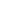#MCR3U - Math Grade 11 is a Course

## MCR3U - Math Grade 11

Started Jan 17, 2021

### Full course description

This course introduces the mathematical concept of the function by extending students’ experiences with linear and quadratic relations. Students will investigate properties of discrete and continuous functions, including trigonometric and exponential functions; represent functions numerically, algebraically, and graphically; solve problems involving applications of functions; investigate inverse functions; and develop facility in determining equivalent algebraic expressions. Students will reason mathematically and communicate their thinking as they solve multi-step problems.